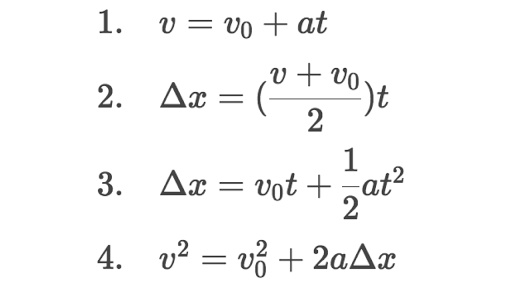# Projectile Motion

I’m trying to make a projectile that does this:
https://i.gyazo.com/a69c9b1286ff51d687e4f2a292902293.mp4

When you aim, the projectile ends up under your cursor, so you have to aim higher to reach your target. That’s what I want. I read Modeling a projectile’s motion - DevForum | Roblox but, EogMoose’s projectile will always travel and land exactly on your mouse.Hit.Position and it will give whatever velocity it needs to reach it in the given time. And when targeting the sky, the projectile basically disappears because of where mouse.Hit.Position is and how fast the velocity is.

In the GIF above, it doesn’t disappear when targeting the sky, and it looks like it travels at a constant speed. If you want a feel of the projectile, you can play it here Marvel: Enhanced - Roblox and test the projectile by pressing R

I messaged the owner of the game and she used BodyVelocity and for the velocity she did:

``````CFrame.new(StartPosition,MousePosition).LookVector * (Velocity number)
``````

Me being new to this kind of math, did this:

My Code
``````tool.Activated:Connect(function()
if not DB then
local comet = CometVFX:Clone()
comet.CFrame = hrp.CFrame
comet.Parent = game.Workspace

local BV = Instance.new('BodyVelocity')
BV.MaxForce = Vector3.new(math.huge,math.huge,math.huge)
BV.Velocity = (CFrame.new(hrp.Position,mouse.Hit.Position).LookVector * 75)
BV.Parent = comet

spawn(function()
DB = false
wait(5)
comet:Destroy()
end)

while wait() do
BV.Velocity = BV.Velocity - Vector3.new(0,2,0) --[makes it curve]
end
end
end)
``````

This code kind of worked, since I had to aim higher to reach the target, but it fell much faster, so when I aimed at the sky, it barely even went up. It looked like this: https://i.gyazo.com/a0eed0bbbde26d424402f11691dd1be5.mp4
I can tell that what I did was very wrong lmao. So, I need help to fix this code. Thanks in advance!

1 Like

You can try using the motion formulas to achieve it.

There would be some information that you already know. The velocity when the projectile reaches the top would be 0, the acceleration would be -workspace.Gravity because it’s in freefall, the start velocity would be already set, and you want to find the distance.3 Likes

FastCast is definitely what you want. It only does not make making projectiles easier, but also allows you to define “gravity” so you can achieve that just by passing a value like that

1 Like

I took physics in school. The math was easy, but applying the concepts was just something else…How would I apply those formulas to code?

1 Like

Something like this might be useful. We learned this formula, and those relative to it, in my engineering class, relating to kinematics and ballistics. Vi is initial velocity, theta represents the angle of elevation, and g, of course, is gravity. The variable x represents the horizontal distance traveled, which I think could help in your case.1 Like

From what I am seeing after closely examing the video it seems it solves a quadratic formula where the vertex is the mouses position in 3d space. I’ve already done the hard for you cause I had to work for this in the past Graphing Calculator
Basically the vertex is 2 times the x value for t and the y is simply the H value. For z It’s the same thing where it’s 2 times the z value for t.

1 Like

There’s nothing wrong with the code except for the usage of body velocity which I find weird, body velocity tends to like to make the projectile go in a straight path and so you would have to use that odd work around to add gravity which is going to cause a memory leak

This piece of code will never end even when BV gets destroyed. Check out the :Destroy() article to properly destroy and cleanup stuff.

``````part:Destroy()
-- Don't do this:
print(part.Name) --> "Hello, world"
``````

So yeah it might as well be better to use an impulse to set a velocity without interfering with the default Roblox gravity.

The main problem is two things, the velocity and gravity values, the velocity is set too low relative to the gravity. This prevents the projectile from going up and causes the extremely low arc as seen in the video. Here is what I tried out to attempt to mimic the above value, by playing around with the velocity and gravity values, increasing the velocity and decreasing the gravity using a body force:

``````local tool = script.Parent
local Players = game:GetService("Players")
local player = Players.LocalPlayer
local mouse = player:GetMouse()

tool.Activated:Connect(function()
local hrp = player.Character.HumanoidRootPart
if not DB then
local comet = Instance.new("Part")
comet.Size = Vector3.new(2,2,2)
comet.CanCollide = false
comet.CFrame = hrp.CFrame
comet.Parent = game.Workspace

local antiGravity = Instance.new("BodyForce")
local antiGravityFactor = 0.9--1 = gravity force counteracted, 0 = no force lol
antiGravity.Force = comet:GetMass()*workspace.Gravity*antiGravityFactor *Vector3.new(0,1,0)
antiGravity.Parent = comet
local appliedVelocity = (CFrame.new(hrp.Position,mouse.Hit.Position).LookVector * 600)
comet:ApplyImpulse(appliedVelocity)

spawn(function()
DB = false
wait(5)
comet:Destroy()
end)
end
end)
``````
2 Likes

This doesn’t relate to the topic, but this could help optimise your script. You could replace spawn() with game.Debris, heres what It’ll look like

``````game.Debris:AddItem(comet, 5) -- Instance, Duration
``````

What this does is basically destroy the part after the specified duration has passed. I’m not quite sure about the “DB = false” though.

1 Like

Also, you can replace this with:

``````game:GetService("RunService").Heartbeat:Connect(function()
BV.Velocity = BV.Velocity - Vector3.new(0,2,0)
end)
``````

I don’t really recommend using while wait()

1 Like

I did not even think of using Body Forces. I tried your code and it actually comes close to what I’m trying to achieve, and I actually understood what you did. But, I will need to understand how to apply physics formulas because it’s very interesting with things like making projectiles. I will mark your response as the solution.